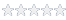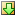# How to Repeat a test multiple times

#### Knowledge Base Article # Q200181

Summary The following example demonstrates how to measure and display the results of similar measurements in a single test loop rather than several individual tests.Login to rate article
In ATEasy, there are many ways to display the results of tests.  The following example demonstrates how to measure and display the results of similar measurements in a single test loop rather than several individual tests. For example, we already have a test that calls an instrument to make a measurement.  We want to measure and display the results eight times.

To achieve this goal, we are going to create a test to make a measurement.  After we take a measuremet we call the RepeatTest() procedure to repeat the test times as required. Finally, we will create a variable to track the number of iterations in a driver public variable m_nTestIteration (initialized to -1).

The test to repeat and make the measurements will be:

DMM Measure(TestResult)
RepeatTest(8) ! add this line at the end of every test that need to be repeated

The procedure to loop would be:

Procedure RepeatTest(nNumberOfIterations) : Void
nNumberOfIterations: Val Short
{
if m_nTestIteration=-1
m_nTestIteration=nNumberOfIterations! start count
endif

m_nTestIteration=m_nTestIteration-1

if m_nCurrentTest=0
return    ! we are done
endif

Test EndEvents Test ! repeat this test
}

Create the module variable:

m_nTestIteration: Short = -1

The result shows that the test 1.1 ran 8 times, taking different measurements each time.From the picture, we can see that the tests numbers were all identical: “001, no matter what iteration was it.  Another problem is that if the last iteration of the test has a PASS status, the program status will show as PASS no matter if one of the test iteration failed. We can add code to include the test iteration in the test number by revising the RepeatTest procedure:

Procedure RepeatTest(nNumberOfIterations) : Void
nNumberOfIterations: Val Short
s: String
i: Short
{
if m_nTestIteration=-1
m_nTestIteration=nNumberOfIterations! start count
endif

m_nTestIteration=m_nTestIteration-1

! display test number - test iteration to the log
s=GetLogString(aLogStringCalcTestStatus)
i=pos(Format(test.Index+1, "000"), s)
SetLogString(Left(s, i)+Format(test.Index+1,"000") + "." + str(nNumberOfIterations-m_nTestIteration) + mid(s, i+len(Format(test.Index+1, "000"))))

! if one test failed fail the program
if TestStatus=FAIL
program.Status=FAIL
endif

if m_nTestIteration=0
return    ! we are done
endif

Test EndEvents Test ! repeat this test
}The ATEasy driver which contains the RepeatTest procedure can be downloadedhere.
Article Date 2/1/2010 , 4/16/2014
Keywords ATEasy

 Login to rate article>